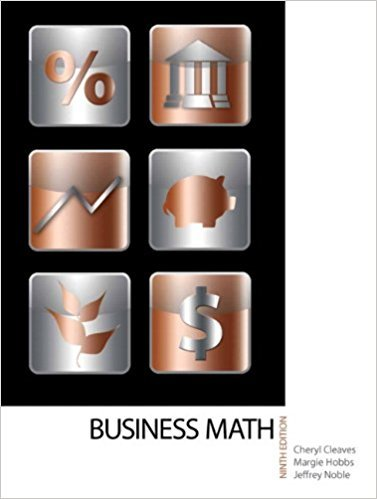×
×

# Solutions for Chapter 19-1: LIFE INSURANCE## Full solutions for Business Math, | 9th Edition

ISBN: 9780135108178Solutions for Chapter 19-1: LIFE INSURANCE

Solutions for Chapter 19-1
4 5 0 370 Reviews
12
5
##### ISBN: 9780135108178

Business Math, was written by and is associated to the ISBN: 9780135108178. This textbook survival guide was created for the textbook: Business Math, , edition: 9. This expansive textbook survival guide covers the following chapters and their solutions. Chapter 19-1: LIFE INSURANCE includes 10 full step-by-step solutions. Since 10 problems in chapter 19-1: LIFE INSURANCE have been answered, more than 17371 students have viewed full step-by-step solutions from this chapter.

Key Math Terms and definitions covered in this textbook
• Column picture of Ax = b.

The vector b becomes a combination of the columns of A. The system is solvable only when b is in the column space C (A).

A sequence of steps (end of Chapter 9) to solve positive definite Ax = b by minimizing !x T Ax - x Tb over growing Krylov subspaces.

• Cramer's Rule for Ax = b.

B j has b replacing column j of A; x j = det B j I det A

• Diagonalization

A = S-1 AS. A = eigenvalue matrix and S = eigenvector matrix of A. A must have n independent eigenvectors to make S invertible. All Ak = SA k S-I.

• Dimension of vector space

dim(V) = number of vectors in any basis for V.

• Fast Fourier Transform (FFT).

A factorization of the Fourier matrix Fn into e = log2 n matrices Si times a permutation. Each Si needs only nl2 multiplications, so Fnx and Fn-1c can be computed with ne/2 multiplications. Revolutionary.

• Four Fundamental Subspaces C (A), N (A), C (AT), N (AT).

Use AT for complex A.

• Fourier matrix F.

Entries Fjk = e21Cijk/n give orthogonal columns FT F = nI. Then y = Fe is the (inverse) Discrete Fourier Transform Y j = L cke21Cijk/n.

• Free columns of A.

Columns without pivots; these are combinations of earlier columns.

• Free variable Xi.

Column i has no pivot in elimination. We can give the n - r free variables any values, then Ax = b determines the r pivot variables (if solvable!).

• Independent vectors VI, .. " vk.

No combination cl VI + ... + qVk = zero vector unless all ci = O. If the v's are the columns of A, the only solution to Ax = 0 is x = o.

• Left inverse A+.

If A has full column rank n, then A+ = (AT A)-I AT has A+ A = In.

• Norm

IIA II. The ".e 2 norm" of A is the maximum ratio II Ax II/l1x II = O"max· Then II Ax II < IIAllllxll and IIABII < IIAIIIIBII and IIA + BII < IIAII + IIBII. Frobenius norm IIAII} = L La~. The.e 1 and.e oo norms are largest column and row sums of laij I.

• Orthogonal matrix Q.

Square matrix with orthonormal columns, so QT = Q-l. Preserves length and angles, IIQxll = IIxll and (QX)T(Qy) = xTy. AlllAI = 1, with orthogonal eigenvectors. Examples: Rotation, reflection, permutation.

• Polar decomposition A = Q H.

Orthogonal Q times positive (semi)definite H.

• Right inverse A+.

If A has full row rank m, then A+ = AT(AAT)-l has AA+ = 1m.

• Row space C (AT) = all combinations of rows of A.

Column vectors by convention.

• Solvable system Ax = b.

The right side b is in the column space of A.

• Spectral Theorem A = QAQT.

Real symmetric A has real A'S and orthonormal q's.

• Sum V + W of subs paces.

Space of all (v in V) + (w in W). Direct sum: V n W = to}.

×